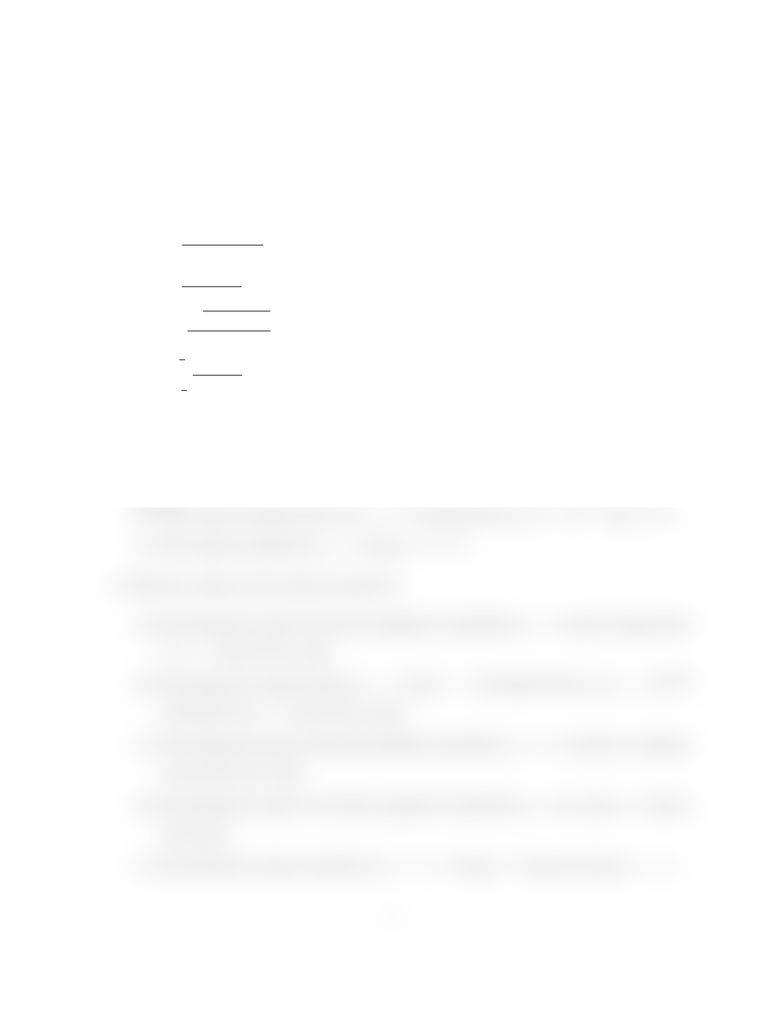Study Guides (390,000)
CA (150,000)
Western (10,000)
MATH (100)
Quiz

# Assignment 10 Solutions.pdf

Department
Mathematics
Course Code
MATH 0110A/B
Professor
Chris Brandl
Study Guide
Quiz

This preview shows half of the first page. to view the full 1 pages of the document.MATH 127 Fall 2012
Practice Assignment (NOT FOR SUBMISSION)
Topics: Integration, area between curves, volumes of revolution
1. Evaluate the following integrals
(a)
Z
4x39x2
x43x3+ 2
dx
(b)
Z
(3x+ 1)2
3x3dx
(c)
Ze
1
p
ln (x2)+1
x
dx
(d)
Zπ
4
π
4
x
cos2(x)
dx
2. Find the area of the indicated region.
(a) The region in the ﬁrst quadrant bounded above by y=x2and y=3x+ 4 and
bounded below by the x-axis.
(b) The region bounded below by y=ex, bounded above by y= 4ex, and x0.
(c) The region bounded by y=x2and y= 2 x2.
3. Find the volume of the object formed by:
(a) Revolving the region in the ﬁrst quadrant bounded by y=exand its tangent line
at x= 1 about the x-axis.
(b) Revolving the region between x= 0 and x= 3 bounded above by y=
x+ 1
and below by y= 1 about the y-axis.
(c) Revolving the region in the ﬁrst quadrant bounded by y= 4xand the coordinate